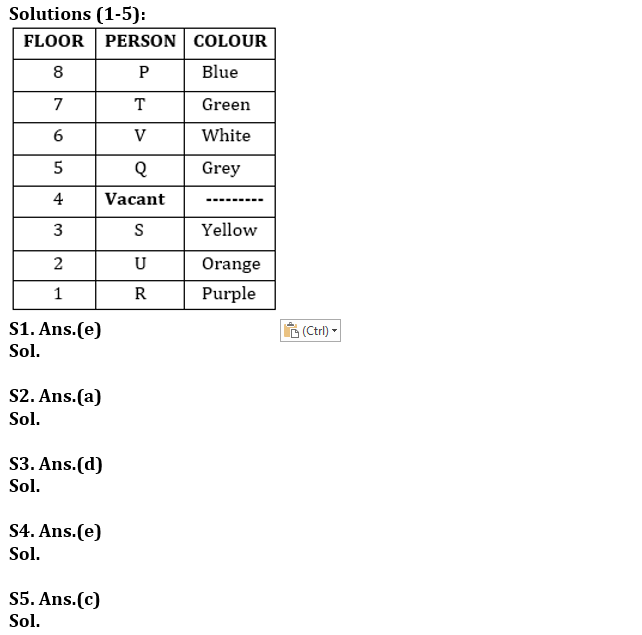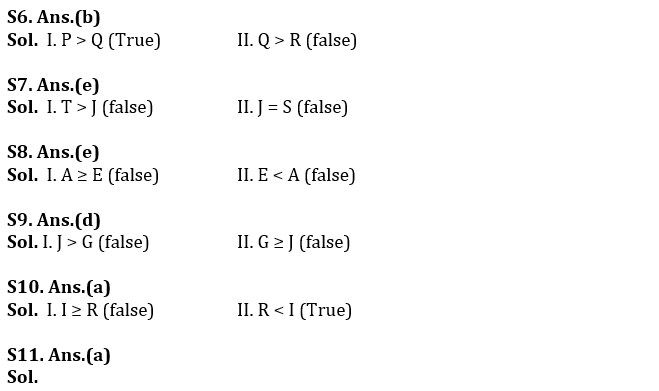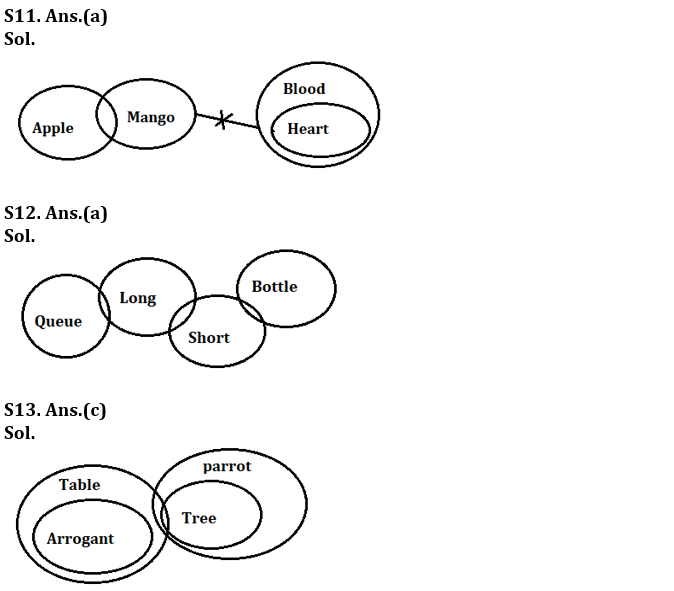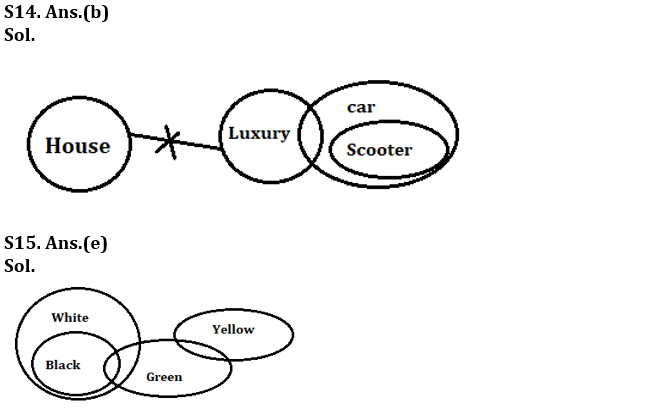Latest Banking jobs   »   Reasoning Ability Quiz For RBI Grade...

# Reasoning Ability Quiz For RBI Grade B/ ECGC PO/ SIDBI Grade A Prelims 2022- 22nd May

Directions (1-5): Read the following information carefully and answer the given questions.
Seven persons P, Q, R, S, T, U and V live on different floors in the same building, the building has eight floors from 1 to 8 in such a way that ground floor is numbered 1 and the top floor is numbered as 8. The top most floor is numbered 8. One floor is vacant. They like different colors- White, Yellow, Orange, Green, Purple, Grey and Blue (but not necessary in the same order).
The one who likes White color lives immediate above the one who likes grey color. The vacant floor is below the 5th floor. Only two persons live between the vacant floor and the one who likes purple color. T likes green color and lives on an odd numbered floor above the vacant floor but not immediate above. Vacant floor is an even numbered floor. The one who likes blue color lives on one of the floors above the floor on which S lives. There are only three floors in between the floor on which V and U live. Q neither likes Yellow nor purple color. U does not live on an odd numbered floor. The one who likes yellow color lives immediate below the vacant floor. Only three persons live between P and the one who likes yellow color. V lives one of the floors above U. There are three persons live between P and S who does not live 1st floor.

Q1. Who lives on the floor just above V?
(a) U
(b) P
(c) the one who likes yellow color
(d) R
(e) the one who likes green color

Q2. How many persons live between S and the one who likes grey color?
(a) None
(b) One
(c) Two
(d) Three
(e) Four

Q3. Which of the following pairs live on the first floor and the topmost floor respectively?
(a) R, the one who likes grey color
(b) T, P
(c) the one who likes orange color, S
(d) the one who likes purple color, P
(e) V, S

Q4. Who among the following lives on the 6th floor?
(a) Q
(b) the one who likes yellow color
(c) P
(d) T
(e) the one who likes White color

Q5. Which of the following combinations is true?
(a) First floor-P
(b) Fourth floor-S
(c) Second floor- the one who likes orange color
(d) Third floor-the one who likes green color
(e) Sixth floor- R

Directions (6-10): In these questions, relationship between different elements is shown in the statements. The statements are followed by conclusions. Study the conclusions based on the given statements and select the appropriate answer. Give answer-

Q6. Statements: S ≤ L ≤ I = P ≥ E > R; L > Q
Conclusions: I. P > Q II. Q > R
(a) If only conclusion II is true
(b) If only conclusion I is true
(c) If both conclusions I and II are true
(d) If either conclusion I or II is true
(e) If neither conclusion I nor II is true

Q7. Statements: G > R ≥ E = A ≤ T ≤ S; D ≤ A ≤ J
Conclusions: I. T > J II. J = S
(a) If only conclusion II is true
(b) If only conclusion I is true
(c) If both conclusions I and II are true
(d) If either conclusion I or II is true
(e) If neither conclusion I nor II is true

Q8. Statements: A ≥ B > C ≤ D, D ≤ E < F
Conclusions: I. A ≥ E II. E < A
(a) If only conclusion II is true
(b) If only conclusion I is true
(c) If both conclusions I and II are true
(d) If either conclusion I or II is true
(e) If neither conclusion I nor II is true

Q9. Statements: G > R ≥ E = A ≤ S; D ≤ A ≤ J
Conclusions: I. J > G II. G ≥ J
(a) If only conclusion II is true
(b) If only conclusion I is true
(c) If both conclusions I and II are true
(d) If either conclusion I or II is true
(e) If neither conclusion I nor II is true

Q10. Statements: S ≤ L ≤ I = P ≥ E > R; L > Q
Conclusions: I. I ≥ R II. R < I
(a) If only conclusion II is true
(b) If only conclusion I is true
(c) If both conclusions I and II are true
(d) If either conclusion I or II is true
(e) If neither conclusion I nor II is true

Directions (11-15): In each of the questions given below some statements are followed by some conclusions. You have to take the given statements to be true even if they seem to be at variance from commonly known facts. Read all the conclusions and then decide which of the given conclusions logically follows from the given statements disregarding commonly known facts.

Q11. Statements: Some Apple are mango. No mango is blood. Only Blood is Heart
Conclusion: I. Some Heart is not Mango
II. All blood can be Apple

(a) If only conclusion I follows
(b) If only conclusion II follows
(c) If either conclusion I or II follows
(d) If neither conclusion I nor II follows
(e) If both conclusions I and II follow

Q12. Statements: Only a few Queue is long. Some Long are Short. Some Short is Bottle
Conclusion: I. All Long can be Short
II. All queue can be Long

(a) If only conclusion I follows
(b) If only conclusion II follows
(c) If either conclusion I or II follows
(d) If neither conclusion I nor II follows
(e) If both conclusions I and II follow

Q13. Statements: All arrogant are table. Some table are tree. All tree are parrot
Conclusion: I. All tree are arrogant
II. Some tree are not arrogant

(a) If only conclusion I follows
(b) If only conclusion II follows
(c) If either conclusion I or II follows
(d) If neither conclusion I nor II follows
(e) If both conclusions I and II follow

Q14. Statements: No house is luxury. Some luxury is car. Only car is Scooter
Conclusions: I. Some scooter can be car
II. Some car is not house

(a) If only conclusion I follows
(b) If only conclusion II follows
(c) If either conclusion I or II follows
(d) If neither conclusion I nor II follows
(e) If both conclusions I and II follow

Q15. Statements: All black are white. Some black are green. Only a few Green are Yellow
Conclusions: I. All green can be white
II. Some yellow can be black

(a) If only conclusion I follows
(b) If only conclusion II follows
(c) If either conclusion I or II follows
(d) If neither conclusion I nor II follows
(e) If both conclusions I and II follow

Solutions#### Congratulations!